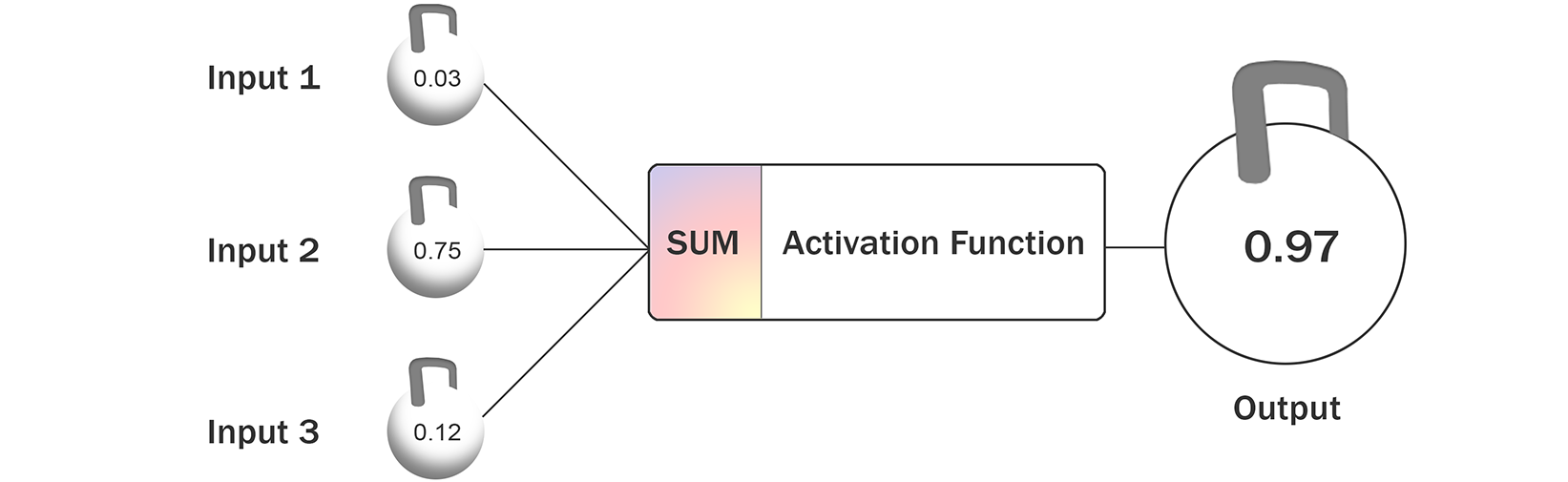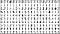# Simple Neural Network StructureMachine Learning at its most basic form — a very simple neural network.

# OK — But How Does It Produce Meaningful Results?

## Recognizing Numbers From A ScreenshotThe same digits written multiple times produce slightly different pattern. Image taken from the JavaScript AI demo located at http://myselph.de/neuralNet.html

# What Does The Trained Set Look Like?

`// The neural network's weights (unit-unit weights, and unit biases)       // training was done in Matlab with the MNIST dataset.// this data is for a 784-200-10 unit, with logistic non-linearity// in the hidden and softmax in the output layer. The input is a// [-1;1] gray level image, background == 1, 28x28 pixels linearized// in column order (i.e. column1(:); column2(:); ...) i-th output// being the maximum means the network thinks the input encodes// (i-1) the weights below showed a 1.92% error rate on the test// data set (9808/10000 digits recognized correctly).let w12 = [[-0.00718674, 0.00941102, -0.0310175, -0.00121102, -0.00978546, -4.65943e-05, 0.0150367, 0.0101846, 0.0482145, 0.00291535, -0.00172736, 0.0234746, 0.0416268, 0.0315077, -0.00252011, 0.0163985, 0.00853601, 0.00836308, 0.00692898, 0.0215552, 0.0540464, 0.0393167, 0.0668207, 0.0232665, 0.031598, 0.0143047, 0.0156885, -0.0269579, -0.00777022, 0.0397823, -0.00825727, 0.0212889, -0.00755215, 0.0353843, 0.0297246, .../* ... Thousands weights more follow ... */`

# Painting Image Input Into Neural Net

`// for visualization/debugging: paint the input to the neural net.         if (document.getElementById('preprocessing').checked == true){    ctx.clearRect(0, 0, canvas.width, canvas.height);    ctx.drawImage(copyCtx.canvas, 0, 0);    for (var y = 0; y < 28; y++) {        for (var x = 0; x < 28; x++) {           var block = ctx.getImageData(x * 10, y * 10, 10, 10);           var newVal = 255 * (0.5 - nnInput[x*28+y]/2);           for (var i = 0; i < 4 * 10 * 10; i+=4) {               block.data[i] = newVal;                               block.data[i+1] = newVal;               block.data[i+2] = newVal;               block.data[i+3] = 255;           }       ctx.putImageData(block, x * 10, y * 10);       }   }}`